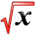# Linear ApproximationsThis article is part of the MathHelp Tutoring Wiki

General Idea:

if f(x) is different to evaluate at some point x, we can use the tangent line at some point close to x, say point a, to approximate f(x)

Recall the equation of the tangent line is,

y=f(a) +f'(a)(x-a)

If we want to evaluate f(x) close to x=a, we can say

f(x)~= f(a)+f'(a)(x-a) ("~=" means almost equal to, but not exactly the same)

The error in doing this is,

E(x)= f(x)-y

E(x)=f(x) -[f(a)+f'(a)(x-a)]

let's take the limit as x->a

lim E(x)= lim f(x)- [f(a)+ f'(a)(x-a)]

x->a x->a

=lim f(x)- lim f(a)- lim f'(a)(x-a)

x->a x->a x->a

=f(a) -f(a)-0

=0

so as x->a, E(x)->0.

Taking f(x)~=f(a)+f'(a)(x-a) is known as as linear approximation or the tangent line approximation.

For example:

Approximate 1.010.3

SOLUTION:

we can write the general equation as

f(x)= x0.3

so f'(x)= 0.3 x-0.7

and in this case, we use a=1, since a=1 is close to 1.01.

using the formula

f(x)~=f(a)+f'(a)(x-a)

~= a0.3 + 0.3a-0.7 (x-a)

~= 1+ 0.3(1.01-1)

~=1+(0.3*0.01)

~=1.003

So (1.01)0.3 ~=1.0038

As we compare to the exact value, ""(1.01)0.3""=1.00298, which is pretty near our approximation.